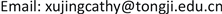﻿ 带真实交易条件的投资组合问题研究及实证分析 Research on Portfolio Optimization with Re-al-Life Transaction Restrictions and Empirical Analysis

Operations Research and Fuzziology
Vol.07 No.04(2017), Article ID:22832,7 pages
10.12677/ORF.2017.74017

Research on Portfolio Optimization with Real-Life Transaction Restrictions and Empirical Analysis

Jing Xu

School of Economics and Management, Tongji University, ShanghaiReceived: Nov. 4th, 2017; accepted: Nov. 17th, 2017; published: Nov. 28th, 2017ABSTRACT

In this paper, we study the theory and empirical analysis of mean-variance model with real transaction restrictions. We introduce the 0-1 variable to the semicontinuous variable and transform the model into an integer programming problem. Based on the idea of quadratic convex reformulation, we propose a new quadratic convex reformulation of research model. By solving the optimal parameters of the new reformulation, the lower bound of the continuous relaxation of the original problem is improved, so as to improve the efficiency of the branch and bound algorithm. Finally, this paper analyzes the stock data of China and validates the efficiency.

Keywords:Mean-Variance Model, Semicontinuous Variable, Quadratic Convex ReformulationCopyright © 2017 by author and Hans Publishers Inc.1. 引言

1952年，美国经济学家Markowitz在其发表的(Portfolio Selection)  文中，第一次从数理经济学的角度揭示了如何通过分散投资以达到降低风险的作用，开创了现代投资分析理论的先河。Markowitz在  中提出了均值–方差模型，用均值和方差分别表示资产的收益和风险，将资产的配置问题转化为一个二次规划问题，即在既定的收益情况下，寻求风险最小的组合，或是给定风险的情况下，寻找收益最大化的投资组合，建立了著名的马科维茨投资组合理论。Sharp对马科维茨均值–方差模型进行了简化，提出了单指数模型，将组合的风险和收益与市场组合联系起来，大大降低了模型的计算量  。Sharp，Lintner和Mossin对马科维茨模型进行了进一步的发展，建立了资本资产定价模型，对证券收益和市场组合收益之间变动的敏感性进行了分析    。经过多位学者的深入研究，马科维兹投资组合理论在应用性等方面得到了极大的提升。随着我国金融体系与世界的接轨，人民币国际化的大趋势，以及外汇、债券、大宗商品、衍生品等金融工具的发展和完善，金融风险管理成为金融学的一个重要课题。如何在这种不确定的环境下进行资本的优化和配置成为机构投资者和普通投资人面临的重要问题，也即投资组合优化问题。

2. 模型构建

$\begin{array}{l}\left(\text{P}\right):\text{min}q\left(x\right)={x}^{\text{T}}Qx\\ \text{s}\text{.t}\text{.}\text{\hspace{0.17em}}{\mu }^{\text{T}}x\ge \rho \\ \text{}\sum _{i=1}^{n}{x}_{i}=1\end{array}$

$\begin{array}{l}\left(\text{P}\right):\text{min}q\left(x\right)={x}^{\text{T}}Qx\\ \text{s}\text{.t}\text{.}\text{\hspace{0.17em}}{\mu }^{\text{T}}x\ge \rho \\ \text{}\sum _{i=1}^{n}{x}_{i}=1\\ \text{}{‖x‖}_{0}\le K\\ \text{}{x}_{i}\in \left\{0\right\}\cup \left[{a}_{i},{b}_{i}\right],i=1,\cdots ,n\end{array}$

3. 模型求解

$\begin{array}{l}\left(\text{P}\right):\text{min}q\left(x\right)={x}^{\text{T}}Qx\\ \text{s}\text{.t}\text{.}\text{\hspace{0.17em}}{\mu }^{\text{T}}x\ge \rho \text{}\text{ }\text{ }\text{}\left(\text{1}\right)\\ \text{}{e}^{\text{T}}x=1\text{}\text{ }\text{}\text{ }\text{\hspace{0.17em}}\text{}\left(\text{2}\right)\\ \text{}{e}^{\text{T}}y\le K\text{}\text{ }\text{}\text{\hspace{0.17em}}\text{ }\text{}\left(\text{3}\right)\\ \text{}{a}_{i}{y}_{i}\le {x}_{i}\le {b}_{i}{y}_{i},i=1,\cdots ,n\text{}\text{\hspace{0.17em}}\text{}\left(4\right)\\ \text{}y\in {\left\{0,1\right\}}^{n}\text{}\text{\hspace{0.17em}}\text{\hspace{0.17em}}\text{}\left(5\right)\end{array}$

$\begin{array}{l}{g}_{\sigma ,\lambda }={\sum }_{i=1}^{n}{\sigma }_{i}\left({y}_{i}^{2}-{y}_{i}\right)+{\sum }_{i=1}^{n}{\lambda }_{i}\left({x}_{i}{y}_{i}-{x}_{i}\right)\\ {f}_{P,N}={\sum }_{i,j=1}^{n}{P}_{ij}\left[-{y}_{i}{y}_{j}+\mathrm{max}\left(0,{y}_{i}+{y}_{j}-1\right)\right]+{\sum }_{i,j=1}^{n}{N}_{ij}\left[{y}_{i}{y}_{j}-\mathrm{min}\left({y}_{i},{y}_{j}\right)\right]\end{array}$

$\begin{array}{l}P\left(\sigma ,\lambda ,P,N\right)\text{}\mathrm{min}\text{}{q}_{\sigma ,\lambda ,P,N}\left(x,y\right)=q\left(x,y\right)+{g}_{\sigma ,\lambda }+{f}_{P,N}\text{}\text{\hspace{0.17em}}\text{\hspace{0.17em}}\text{\hspace{0.17em}}\text{\hspace{0.17em}}\text{}\text{\hspace{0.17em}}\text{\hspace{0.17em}}\text{\hspace{0.17em}}\text{\hspace{0.17em}}\text{\hspace{0.17em}}\text{}\text{\hspace{0.17em}}\text{\hspace{0.17em}}\text{\hspace{0.17em}}\text{\hspace{0.17em}}\text{\hspace{0.17em}}\text{}\left(\text{6}\right)\\ \text{}\text{\hspace{0.17em}}\text{\hspace{0.17em}}\text{s}\text{.t}\text{.}\text{\hspace{0.17em}}\text{\hspace{0.17em}}\left(1\right),\left(2\right),\left(3\right),\left(4\right),\left(5\right)\end{array}$

$\begin{array}{l}P\left(\sigma ,\lambda ,P,N\right)\text{}\mathrm{min}\text{}{q}_{\sigma ,\lambda ,P,N}\left(x,y\right)=q\left(x,y\right)+{g}_{\sigma ,\lambda }+{\sum }_{i,j=1}^{n}{P}_{ij}\left[-{y}_{i}{y}_{j}+{u}_{ij}\right]\end{array}$ $+{\sum }_{i,j=1}^{n}{N}_{ij}\left[{y}_{i}{y}_{j}+{v}_{ij}\right]$
$\text{}\text{s}\text{.t}\text{.}\text{}{\mu }^{\text{T}}x\ge \rho ,{e}^{\text{T}}x=1,{e}^{\text{T}}y\le K$
$\text{}{a}_{i}{y}_{i}\le {x}_{i}\le {b}_{i}{y}_{i},i=1,\cdots ,n,{y}_{i}\in \left\{0,1\right\}$
$\text{}{u}_{ij}\ge 0,{u}_{ij}\ge {y}_{i}+{y}_{j}-1,i,j=1,\cdots ,n$
$\text{}{v}_{ij}\ge -{y}_{i},{v}_{ij}\ge -{y}_{j},i,j=1,\cdots ,n$

$\begin{array}{l}\left(\text{CP}\right)\text{}\mathrm{max}\text{}v\left(P\left(\sigma ,\lambda ,P,N\right)\right)\\ \text{}\text{s}\text{.t}\text{.}\text{\hspace{0.17em}}\left(\sigma ,\lambda \right)\in {R}^{n}×{R}^{n},\left(P,N\right)\in {S}^{n}×{S}^{n},{P}_{ij}\ge 0,{N}_{ij}\ge 0,i,j=1,\cdots ,n\text{}\text{\hspace{0.17em}}\text{\hspace{0.17em}}\text{\hspace{0.17em}}\text{\hspace{0.17em}}\text{\hspace{0.17em}}\text{}\text{\hspace{0.17em}}\text{\hspace{0.17em}}\text{\hspace{0.17em}}\text{\hspace{0.17em}}\text{\hspace{0.17em}}\text{}\text{\hspace{0.17em}}\text{\hspace{0.17em}}\text{\hspace{0.17em}}\text{}\text{\hspace{0.17em}}\text{\hspace{0.17em}}\left(\text{7}\right)\\ \text{}\left(\begin{array}{cc}Q& \frac{1}{2}\text{diag}\left(\lambda \right)\\ \frac{1}{2}\text{diag}\left(\lambda \right)& \text{diag}\left(\sigma \right)+N-P\end{array}\right)\succcurlyeq 0\text{}\text{ }\text{}\text{\hspace{0.17em}}\text{\hspace{0.17em}}\text{\hspace{0.17em}}\text{}\text{\hspace{0.17em}}\text{\hspace{0.17em}}\text{\hspace{0.17em}}\text{\hspace{0.17em}}\text{\hspace{0.17em}}\text{\hspace{0.17em}}\text{\hspace{0.17em}}\text{\hspace{0.17em}}\text{\hspace{0.17em}}\text{\hspace{0.17em}}\text{\hspace{0.17em}}\text{\hspace{0.17em}}\text{ }\left(8\right)\end{array}$

$\begin{array}{l}\left(\text{SDP}\right)\text{}\mathrm{max}\text{}\tau \\ \text{}\text{s}\text{.t}\text{.}\left(\begin{array}{ccc}Q& \frac{1}{2}\text{diag}\left(\lambda \right)& \frac{1}{2}\left(-\lambda +\eta e+\beta -\alpha \right)\\ \frac{1}{2}\text{diag}\left(\lambda \right)& \text{diag}\left(\sigma \right)+N-P& \frac{1}{2}O\\ \frac{1}{2}\left(-\lambda +\eta e+\beta -\alpha \right)& \frac{1}{2}{O}^{T}& -\eta -Ks-{e}^{\text{T}}\pi -{e}^{\text{T}}He-\tau \end{array}\right)\succcurlyeq 0\\ \text{}\kappa \in {R}_{+},\eta \in {R}_{+},s\in {R}_{+}\\ \text{}\left(\alpha ,\beta ,\zeta ,\pi \right)\in {R}_{+}^{n}×{R}_{+}^{n}×{R}_{+}^{n}×{R}_{+}^{n}\\ \text{}\left(H,T,M,R\right)\in {S}^{n}×{S}^{n}×{S}^{n}×{S}^{n}\\ \text{}{H}_{ij}\ge 0,{T}_{ij}\ge 0,{M}_{ij}\ge 0,{R}_{ij}\ge 0,i,j=1,\cdots ,n\\ \text{}P=H+T\\ \text{}N=M+R\end{array}$

$O=-\sigma +se+\pi +\text{diag}\left(a\right)\alpha -\text{diag}\left(b\right)\beta +\sum _{i,j=1}^{n}\left[\left({H}_{ij}-{M}_{ij}\right){e}_{i}+\left({H}_{ij}-{R}_{ij}\right){e}_{j}\right]$

$\left({\sigma }^{*},{\lambda }^{*},{P}^{*},{N}^{*},{\alpha }^{*},{\beta }^{*},{\zeta }^{*},{\pi }^{*},{H}^{*},{T}^{*},{M}^{*},{R}^{*}\right)$ 为(SDP)的最优解，则 $\left({\sigma }^{*},{\lambda }^{*},{P}^{*},{N}^{*}\right)$ 为(CP)的最优解，且 $v\left(\text{SDP}\right)=v\left(\text{CP}\right)=v\left(\text{P}\right)$

$L\left(x,y,u,v\right)$ 来表示 $P\left(\sigma ,\lambda ,P,N\right)$ 的拉格朗日对偶形式，对偶系数的范围分别为： $\left(\kappa ,\eta ,s\right)\in {R}_{+}×{R}_{+}×{R}_{+}$$\left(\alpha ,\beta ,\zeta ,\pi \right)\in {R}_{+}^{n}×{R}_{+}^{n}×{R}_{+}^{n}×{R}_{+}^{n}$$\left(H,T,M,R\right)\in {S}^{n}×{S}^{n}×{S}^{n}×{S}^{n}$ 。用 $\omega$ 表示 $\kappa ,\eta ,s,\alpha ,\beta ,\zeta ,\pi ,H,T,M,R$ 组成的向量，故而 $P\left(\sigma ,\lambda ,P,N\right)$ 的拉格朗日对偶问题可以表示为：

$\mathrm{max}\left\{\mathrm{min}L\left(x,y,u,v\right)|\omega \ge 0\right\}$

$\begin{array}{l}L\left(x,y,u,v\right)={x}^{\text{T}}Qx+\sum _{i=1}^{n}{\sigma }_{i}\left({y}_{i}^{2}-{y}_{i}\right)+\sum _{i=1}^{n}{\lambda }_{i}{x}_{i}\left({y}_{i}-1\right)+\sum _{i,j=1}^{n}\left({N}_{ij}-{P}_{ij}\right){y}_{i}{y}_{j}\\ \text{\hspace{0.17em}}\text{\hspace{0.17em}}\text{\hspace{0.17em}}\text{\hspace{0.17em}}\text{\hspace{0.17em}}\text{\hspace{0.17em}}\text{\hspace{0.17em}}\text{\hspace{0.17em}}\text{\hspace{0.17em}}\text{\hspace{0.17em}}\text{\hspace{0.17em}}\text{\hspace{0.17em}}\text{\hspace{0.17em}}\text{\hspace{0.17em}}\text{\hspace{0.17em}}\text{\hspace{0.17em}}\text{\hspace{0.17em}}\text{\hspace{0.17em}}+\sum _{i,j=1}^{n}\left({P}_{ij}{u}_{ij}+{N}_{ij}{v}_{ij}\right)-\kappa \left({\mu }^{\text{T}}x-\rho \right)+\eta \left({e}^{\text{T}}x-1\right)+s\left({e}^{\text{T}}y-K\right)\\ \text{\hspace{0.17em}}\text{\hspace{0.17em}}\text{\hspace{0.17em}}\text{\hspace{0.17em}}\text{\hspace{0.17em}}\text{\hspace{0.17em}}\text{\hspace{0.17em}}\text{\hspace{0.17em}}\text{\hspace{0.17em}}\text{\hspace{0.17em}}\text{\hspace{0.17em}}\text{\hspace{0.17em}}\text{\hspace{0.17em}}\text{\hspace{0.17em}}\text{\hspace{0.17em}}\text{\hspace{0.17em}}\text{\hspace{0.17em}}\text{\hspace{0.17em}}+\sum _{i=1}^{n}{\alpha }_{i}\left({a}_{i}{y}_{i}-{x}_{i}\right)+\sum _{i=1}^{n}{\beta }_{i}\left({x}_{i}-{b}_{i}{y}_{i}\right)-{\xi }^{\text{T}}y+{\pi }^{\text{T}}\left(y-e\right)\\ \text{\hspace{0.17em}}\text{\hspace{0.17em}}\text{\hspace{0.17em}}\text{\hspace{0.17em}}\text{\hspace{0.17em}}\text{\hspace{0.17em}}\text{\hspace{0.17em}}\text{\hspace{0.17em}}\text{\hspace{0.17em}}\text{\hspace{0.17em}}\text{\hspace{0.17em}}\text{\hspace{0.17em}}\text{\hspace{0.17em}}\text{\hspace{0.17em}}\text{\hspace{0.17em}}\text{\hspace{0.17em}}\text{\hspace{0.17em}}\text{\hspace{0.17em}}+\sum _{i,j=1}^{n}{H}_{ij}\left({y}_{i}+{y}_{j}-1-{u}_{ij}\right)-\sum _{i,j=1}^{n}{T}_{ij}{u}_{ij}+\sum _{i=1}^{n}{M}_{ij}\left(-{y}_{i}-{v}_{ij}\right)+\sum _{i,j=1}^{n}{R}_{ij}\left(-{y}_{i}-{v}_{ij}\right)\\ \text{}={L}_{1}\left(x,y\right)+{L}_{2}\left(u,v\right)\\ \text{}=\left\{\begin{array}{l}{L}_{1}\left(x,y\right)\text{\hspace{0.17em}}\text{\hspace{0.17em}}\text{\hspace{0.17em}}\text{\hspace{0.17em}}\text{\hspace{0.17em}}当P-H-K=0且N-M-R=0\\ -\infty \text{\hspace{0.17em}}\text{\hspace{0.17em}}\text{\hspace{0.17em}}\text{\hspace{0.17em}}\text{\hspace{0.17em}}\text{\hspace{0.17em}}\text{\hspace{0.17em}}\text{\hspace{0.17em}}\text{\hspace{0.17em}}\text{\hspace{0.17em}}\text{\hspace{0.17em}}\text{\hspace{0.17em}}\text{\hspace{0.17em}}\text{\hspace{0.17em}}\text{\hspace{0.17em}}\text{\hspace{0.17em}}\text{\hspace{0.17em}}\text{\hspace{0.17em}}\text{\hspace{0.17em}}\text{\hspace{0.17em}}\text{\hspace{0.17em}}\text{\hspace{0.17em}}\text{\hspace{0.17em}}\text{\hspace{0.17em}}\text{\hspace{0.17em}}其他\end{array}\end{array}$

$\begin{array}{l}{L}_{1}\left(x,y\right)=\left(\begin{array}{cc}{x}^{\text{T}}& {y}^{\text{T}}\end{array}\right)\left(\begin{array}{cc}Q& \frac{1}{2}\text{diag}\left(\lambda \right)\\ \frac{1}{2}\text{diag}\left(\lambda \right)& \text{diag}\left(\sigma \right)+N-P\end{array}\right)\left(\begin{array}{c}x\\ y\end{array}\right)+{\left(-\lambda -\kappa \mu +\eta e-\alpha +\beta \right)}^{\text{T}}x\\ \text{}+{\left\{-\sigma +se-\zeta +\pi +\text{diag}\left(a\right)\alpha -\text{diag}\left(b\right)\beta +\sum _{i,j=1}^{n}\left[\left({H}_{ij}-{M}_{ij}\right){e}_{i}+\left({H}_{ij}-{R}_{ij}\right){e}_{j}\right]\right\}}^{\text{T}}y\\ \text{}+\rho \kappa -\eta -Ks-{e}^{\text{T}}\pi -{e}^{\text{T}}He\\ {L}_{2}\left(u,v\right)=\text{tr}\left[\left(P-H-K\right){u}^{\text{T}}\right]+\text{tr}\left[\left(N-M-R\right){v}^{\text{T}}\right]\end{array}$

$\left(\begin{array}{ccc}Q& \frac{1}{2}\text{diag}\left(\lambda \right)& \frac{1}{2}\left(-\lambda +\eta e+\beta -\alpha \right)\\ \frac{1}{2}\text{diag}\left(\lambda \right)& \text{diag}\left(\sigma \right)+N-P& \frac{1}{2}O\\ \frac{1}{2}\left(-\lambda +\eta e+\beta -\alpha \right)& \frac{1}{2}{O}^{T}& -\eta -Ks-{e}^{\text{T}}\pi -{e}^{\text{T}}He-\tau \end{array}\right)\succcurlyeq 0$

4. 数值实验

5. 结论

Research on Portfolio Optimization with Re-al-Life Transaction Restrictions and Empirical Analysis[J]. 运筹与模糊学, 2017, 07(04): 163-169. http://dx.doi.org/10.12677/ORF.2017.74017

1. 1. Markowitz, H. (1952) Portfolio Selection. The Journal of Finance, 7, 77-91.

2. 2. Sharpe, W.F. (1963) A Simplified Model For Portfolio Analysis. Management Science, 9, 277-293. https://doi.org/10.1287/mnsc.9.2.277

3. 3. Sharpe, W.F. (1964) Capital Asset Price: A Theory of Market Equilibrium under Conditions of Risk. Journal of Finance, 19, 425-442.

4. 4. Linter, J. (1965) The Valuation of Risk Assets and the Selection of Risky Investments in Stock Porfolios and Capital Budgets. Review of Economics and Statistics, 47, 13-37. https://doi.org/10.2307/1924119

5. 5. Mossin, J. (1966) Equilibrium in a Capital Asset Market. Econometrica, 34,768-783. https://doi.org/10.2307/1910098

6. 6. 孙小玲, 李端. 整数规划新进展[J]. 运筹学学报, 2014, 18(1): 39-68.

7. 7. Anatreicher, K. (2009) Semidefinite Programming versus the Reformulation-Linearization Technique for Nonconvex Quadratically Constrained Quadratic Programming. Journal of Global Optimization, 43, 471-484. https://doi.org/10.1007/s10898-008-9372-0

8. 8. Billionnet, A., Elloumi, S. and Plateau, M. (2009) Improving the Performance of Standard Solvers for Quadratic 0-1 Programs by a Tight Convex Reformulation: The QCR Method. Discrete Applied Mathematics, 157, 1185-1197. https://doi.org/10.1016/j.dam.2007.12.007